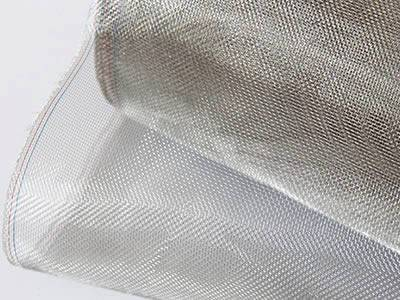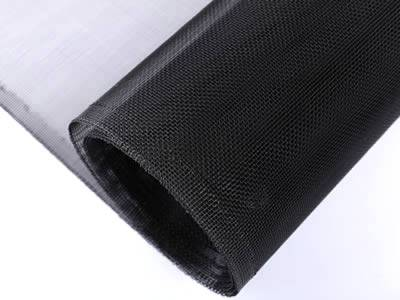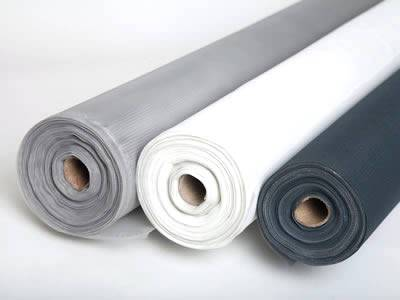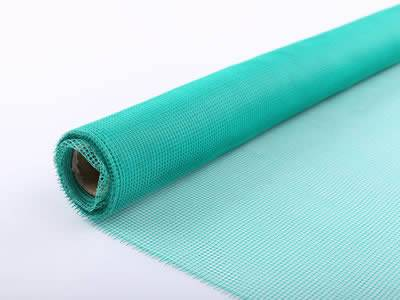# Weight Calculation Method for All Sorts of Window Screens

• Stainless steel window screen weight calculation formula:
• Weight (kg/roll) = 1/2 × wire diameter × wire diameter × mesh × length × width.
• Weight (kg per square meter) = 1/2 × wire diameter × wire diameter × mesh.
• Iron wire window screen weight calculation formula:
• Weight (kg/roll) = 1/2 × wire diameter × wire diameter × mesh × length × width.
• Weight (kg per square meter) = 1/2 × wire diameter × wire diameter × mesh.
• Aluminum alloy window screen weight calculation formula:
• Weight (kg/roll) = 1/2 × wire diameter × wire diameter × mesh × length × width × 0.3.
• Weight (kg per square meter) = 1/2 × wire diameter × wire diameter × mesh × 0.3.
• Plastic window screen weight calculation formula:
• Weight (kg/roll) = 1/2 × (wire diameter × wire diameter × mesh × length × width) / 6.78.
• Weight (kg per square meter) = 1/2 × wire diameter × wire diameter × mesh / 6.78.
• Fiberglass window screen weight calculation formula:
• Weight (kg/roll) = 1/2 × (wire diameter × wire diameter × mesh × length × width) / 4.5.
• Weight (kg per square meter) = 1/2 × (wire diameter × wire diameter × mesh) / 4.5.WC-01: Stainless steel insect screen.WC-02: Aluminum insect screen.WC-03: Plastic window screen.WC-04: Fiberglass window screen.

Inquiry for Our Product

Shandong Xingying Environmental Energy Technology Co. LTD
E-mail: sales@goldoxwiremesh.com

When you contact us, please provide your detail requirements. That will help us give you a valid quotation.

Full Name *
Country Code * + Phone Number *